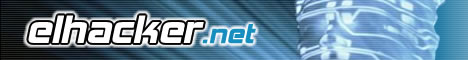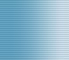Bienvenido(a), Visitante. Por favor Ingresar o Registrarse ¿Perdiste tu email de activación?.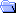Foro de elhacker.net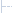Informática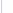ElectrónicaReusing registers in VHDL FSM 0 Usuarios y 1 Visitante están viendo este tema.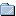Autor Tema: Reusing registers in VHDL FSM  (Leído 3,158 veces)
Darian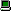Desconectado

Mensajes: 1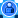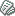Reusing registers in VHDL FSM « en: 3 Julio 2019, 17:22 »

Hello,

I need to write a Finite State Machine (FSM) in VHDL code and  want to have several computations being processed at the same time (a standard pipeline). In every state I have several operations to be calculated and I employ registers for the result of each one. I strongly need to reuse these registers, for example: Register 1 is filled in State 1 (as a result of a multiplication) and it is used in the State 2 and State 3 (as parameter of other operations), then in the State 4, I want to save a new operation result (another multiplication) in Register 1 reusing it.

My code works in Simulation in Xilinx Vivado 2019, but when I implement the desing in a real FPGA (Basys 3 Artix-7) it doesn't work. I realized that the problem is that the correct values are not saved when I reuse the registers. Sometimes, the first time I reuse them, they keep the correct value, but already in the second reuse in later FSM states, the stored values are not correct, I mean, they do not correspond to the result of the operation that I am trying to save in the register.

Next, an example of my FSM design:

Código
`LIBRARY IEEE;USE IEEE.std_logic_1164.all;USE IEEE.numeric_std.ALL; ENTITY test1_arith ISGENERIC (    ap_bit_width : positive := 4;    ap_latency : positive := 2);PORT (    I1 : IN STD_LOGIC_VECTOR(ap_bit_width - 1 downto 0);    I2 : IN STD_LOGIC_VECTOR(ap_bit_width - 1 downto 0);    I3 : IN STD_LOGIC_VECTOR(ap_bit_width - 1 downto 0);    O1 : OUT STD_LOGIC_VECTOR(ap_bit_width - 1 downto 0);    ap_clk : IN STD_LOGIC;    ap_rst : IN STD_LOGIC;    ap_start : IN STD_LOGIC;    ap_done : OUT STD_LOGIC;    ap_idle : OUT STD_LOGIC;    ap_ready : OUT STD_LOGIC);END; ARCHITECTURE test1_arith_arch OF test1_arith IS    ATTRIBUTE CORE_GENERATION_INFO : STRING;    ATTRIBUTE CORE_GENERATION_INFO OF test1_arith_arch : ARCHITECTURE IS "Test,VHDLbyMOEA,{HLS_SYN_LAT=2}";    CONSTANT ap_const_logic_1 : STD_LOGIC := '1';    CONSTANT ap_const_logic_0 : STD_LOGIC := '0';    TYPE state IS (state_1,state_2,state_3);    SIGNAL state_present: state;    SIGNAL state_future: state;     SIGNAL Flag: Integer:=0;     --Signal RF : STD_LOGIC_VECTOR_array;    FUNCTION ALU ( Op: IN integer range 0 TO 23;     A, B: IN STD_LOGIC_VECTOR (ap_bit_width - 1 downto 0) )    RETURN std_logic_vector is variable Result : std_logic_vector(ap_bit_width - 1 downto 0);             variable A_int: Integer:=0;    variable B_int: Integer:=0;    variable Result_int: Integer:=0;    begin    A_int := to_integer(unsigned(A));    B_int := to_integer(unsigned(B));    With Op Select Result_int:=        to_integer(unsigned(NOT A)) When 0,        to_integer(unsigned(A AND B)) When 1,        to_integer(unsigned(A OR B)) When 2,        to_integer(unsigned(A NAND B)) When 3,        to_integer(unsigned(A NOR B)) When 4,        to_integer(unsigned(A XOR B)) When 5,        to_integer(unsigned(A XNOR B)) When 6,        (A_int + B_int) When 7,        (A_int - B_int) When 8,        (A_int * B_int) When 9,        (A_int / B_int) When 10,        ABS(A_int) When 11,        (A_int ** B_int) When 12,        (A_int MOD B_int) When 13,        to_integer(unsigned(A) & unsigned(B)) When 14,        to_integer(unsigned(A) SLL B_int) When 15,        to_integer(unsigned(A) SRL B_int) When 16,        to_integer(unsigned(A) SLA B_int) When 17,        to_integer(unsigned(A) SRA B_int) When 18,        to_integer(unsigned(A) ROL B_int) When 19,        to_integer(unsigned(A) ROR B_int) When 20,        to_integer(unsigned(A) & unsigned(B)) When 21,        to_integer(unsigned(A) & unsigned(B)) When 22,                 0   When others;    return STD_LOGIC_VECTOR (TO_UNSIGNED (Result_int, (ap_bit_width)));    END FUNCTION;     SHARED VARIABLE R1:std_logic_vector(ap_bit_width - 1 downto 0);          BEGIN     OP_FSM : PROCESS (state_present)      BEGIN     CASE state_present IS     WHEN state_1=>     R1 := ALU(Op => 7 ,A => I1,B => I2);    Flag<=1;    IF (Flag=1) THEN    state_future <= state_2;    END IF;     WHEN state_2=>     R1:= ALU(Op => 7 ,A => R1, B => I3);    Flag<=2;    IF (Flag=2) THEN    state_future <= state_3;    END IF;     WHEN state_3=>     O1<= ALU(Op => 7 ,A => R1,B => "0001");    Flag<=3;    IF (Flag=3) THEN    state_future <= state_1;    END IF;    END CASE;    END PROCESS OP_FSM;     CLK_FSM : PROCESS (ap_clk)    BEGIN    IF (ap_clk = '1' AND ap_clk'EVENT) THEN    state_present <= state_future;    END IF;    END PROCESS CLK_FSM; END test1_arith_arch;`

In this case, I want to reuse R1 and it works well in Simulation with Xilinx Vivado (1 + 4 + 0 + 1 = 6).

Unfortunately, in the Basys 3 FPGA Artix-7 I don't get the correct results. In the Case 10 in a FPGA, it should get 6 (1 + 4 + 0 + 1) as result, but it gets 14 instead.

In the tests that I have been doing I realized that it works better when before assigning a new value in the registry the value of the record is made zero before reassigning a value, for example:

Código
`WHEN state_3=>     R4<="0000"    IF( R4 = "0000") then    R4<= ALU(Op => 7 ,A=> R2,B=> R3, C =>"0000");    Flag <=3;    IF (Flag =3) THEN    state_future <= state_4;END IF;END IF;`

Using this form I can reuse a register once, the second time I want to reassign a value to the register, incorrect values are shown in the output.

I declarated the registers as SHARED VARIABLE and SIGNALS and I have the same problem with both.

I appreciate any suggestion or idea, thanks a lot.En línea

Ir a:

 Mensajes similares Asunto Iniciado por Respuestas Vistas Último mensajeEvitar que te borren los Debug registers en un debugger ring3 ASM biribau 3 2,584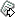18 Junio 2009, 16:41 por biribau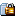VHDL Electrónica ukol 6 8,5807 Enero 2019, 01:30 por mochileraProgramacion en VHDL Programación General Fox_Neo 1 3,21410 Octubre 2013, 17:44 por Fox_Neo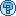VGA en VHDL Foro Libre joan.ayala 0 73823 Mayo 2014, 22:52 por joan.ayalaVHDL Electrónica carlospulido3 2 3,40027 Enero 2019, 16:08 por andresiniesta